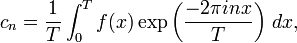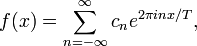# Fourier series

In mathematics, the Fourier series, named after Joseph Fourier (1768—1830), of a complex-valued periodic function f of a real variable, is an infinite series$\sum_{n=-\infty}^\infty c_n e^{2\pi inx/T}$

defined by$c_n = \frac{1}{T} \int_0^T f(x) \exp\left(\frac{-2\pi inx}{T}\right)\,dx,$

where T is the period of f.

In what sense it may be said that this series converges to f(x) is a somewhat delicate question. However, physicists being less delicate than mathematicians in these matters, simply write$f(x) = \sum_{n=-\infty}^\infty c_n e^{2\pi inx/T},$

and usually do not worry too much about the conditions to be imposed on the arbitrary function f(x) of period T for this expansion to converge to it.Some content on this page may previously have appeared on Citizendium.